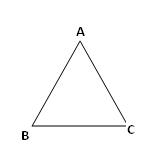you are here->home->Physics->Class 11->Spherometer
Spherometer

# Materials Required

• Spherometer
• Glass strip
• Concave surface
• Plane glass slab
• Glass strip
• A sheet of paper
• A ruler
• Pencil

# Real Lab Procedure

## To find the distance between the legs of the Spherometer

1. Raise the central screw of the spherometer and press the spectrometer gently on a sheet of paper so as to get the pin pricks of the three legs. Mark these pricks as A, B and C.
2. Measure the distance between the pricks by joining the points as to form a triangle.
3. Note these distances (AB, BC, and AC) on the paper and take their mean as l.

## To find the Least count of the Spherometer

1. Note one pitch scale division on the pitch scale or vertical scale.
2. Take 5 full rotations on the central screw.
3. Measure the distance moved by the screw.
4. Hence, Pitch = Distance moved /number of full rotations.
5. Then## To find the radius curvature of the spherical surface

1. Raise the screw sufficiently upwards.
2. Place the spherometer on the concave surface so that its three legs rest on it.
3. Gently turn the screw downwards till the screw tip just touches the concave surface.
4. Note the reading of the circular disc scale which is in line with the vertical (pitch) scale. Note this reading as 'a', which will act as reference.
5. Remove the spherometer from over the concave surface and place it over a large size glass slab.
6. Turn the screw down wards and count the number of complete rotations made by the disc (one rotation becomes complete when the reference reading crosses past the pitch scale.)
7. Continue till the tip of the screw just touches the plane surface of the glass slab.
8. Note the reading of the circular scale which is finally in line with the vertical (pitch) scale. Note this reading as 'b'.
9. Find the number of circular (disc) scale division in the last incomplete rotation.
10. Now find total reading using the relation equation 1.
11. Repeat steps 3 to 9, three times .Record the observation in tabular form.
12. Calculate the radius of curvature of the given concave surface using the equation 2.

## To find the thickness of the glass plate

1. Raise the screw sufficiently upwards.
2. Place the spherometer on the glass strip so that it rests between its three legs.
3. Repeat the above steps 3 to 9, three times .Record the observation in a tabular form.
4. Find total reading using the relation equation 1.

# Simulator Procedure (as performed through the Online Labs)

1. Click on the object shown on the left hand menu, to measure its thickness
2. Tighten the screw by clicking on the respective arrows (left / right) on the screw, until it touches the object.
3. Note the reading on pitch scale, the circular disc and note it down as the reference variable.
4. Again click on the object on the left hand menu to remove it from under the spherometer.
5. Tighten the screw by clicking on the respective arrows (left / right) on the screw, until it touches the glass slab.
6. Note down the complete rotations on the pitch scale and note the reading on circular disc for fractional rotation.
7. Based on the selected object;
• Calculate the radius of curvature if it is the spherical surface using the equation 2.
• calculate the thickness it it is the glass strip using the equation 1.
8. Note down the reading in the text box.
9. Click on the check button to find if the answer is correct.
10. To redo the experiment, click on the 'Reset' button.

# Observations

## 1.Distance between two legs of the spherometer

In  triangle ABC marked by the legs of the spherometer

AB = ------cm

BC = ------cm

AC = ------cmMean value of  l is,= ----cm

## 2.Least count of Spherometer

1 pitch scale division = 1mm

Number of full rotations given to screw = 5

Distance moved by the screw=5mm

Hence pitch,Number of divisions on circular scale = 100

Hence least count=Object placed Circular scale reading No of complete rotation on plane glass sheet(n) No of disc scale divisions in incomplete rotation X=(a-b) or (100+a)-b Total reading = (n x p) +(x x l.c)mm On concave surface(initial) On plane glass sheet(final) Glass strip t = Concave surface h =

# Calculation

The thickness of the glass plate, t = --------------- mm = --------------------m .

Mean value of h = -----------------------mm.

Radius of curvature of the spherical surface= ---------------cm=................m

# Result

The thickness of glass strip =  ------------m

The radius of curvature of the given concavesurface = -----------------m